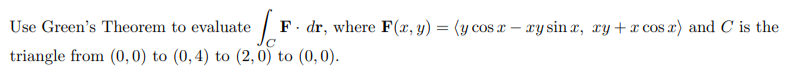# Use Green's Theorem to evaluate | F. dr, where F(x, y) = (y cos x – xy sin r, ry + cos r) and C is the %3D triangle from (0,0) to (0,4) to (2,0) to (0,0).

Question

Could you help me with this question? I'm not sure how to solve for the values. I'd appreciate the help.

Here is the question again [Use Green’s Theorem to evaluate ZC F · dr, where F(x, y) = hy cos x − xy sin x, xy + x cos xi and C is the triangle from (0, 0) to (0, 4) to (2, 0) to (0, 0).]help_outlineImage TranscriptioncloseUse Green's Theorem to evaluate | F. dr, where F(x, y) = (y cos x – xy sin r, ry + cos r) and C is the %3D triangle from (0,0) to (0,4) to (2,0) to (0,0). fullscreen

1 Rating

### Want to see this answer and more?

Experts are waiting 24/7 to provide step-by-step solutions in as fast as 30 minutes!*

*Response times may vary by subject and question complexity. Median response time is 34 minutes for paid subscribers and may be longer for promotional offers.
Tagged in
Math
Calculus

### Applications of Integration